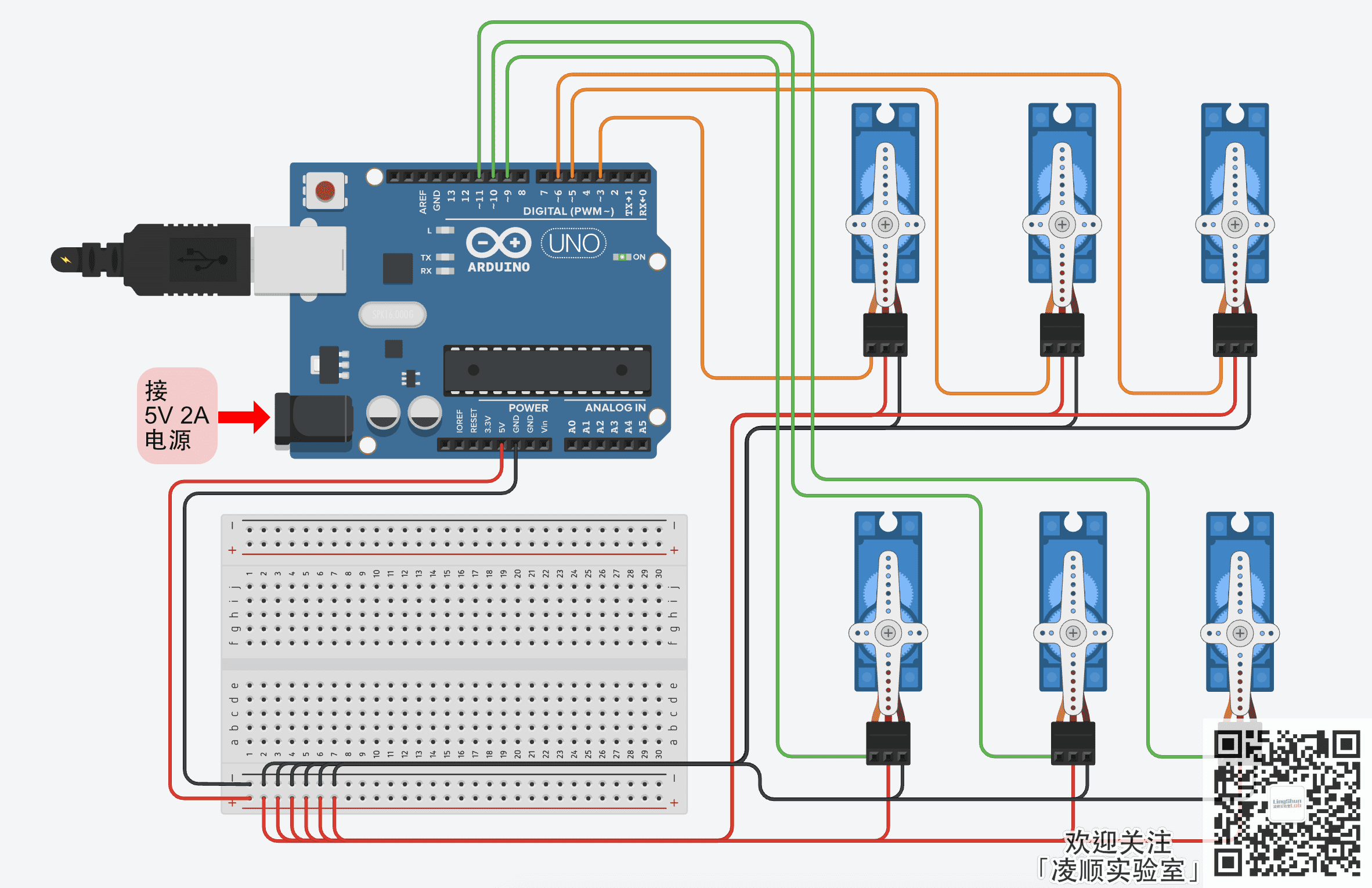# Arduino Uno 同时控制多路舵机

Arduino Uno x1

5V2A电源 x1

## 接线方式

Arduino Uno 引脚 <---> 舵机引脚_1
5V <-> VCC（红色）
GND <-> GND（黑色或褐色）
3 <-> 信号线（橙色）
Arduino Uno 引脚 <---> 舵机引脚_2
5V <-> VCC（红色）
GND <-> GND（黑色或褐色）
5 <-> 信号线（橙色）
Arduino Uno 引脚 <---> 舵机引脚_3
5V <-> VCC（红色）
GND <-> GND（黑色或褐色）
6 <-> 信号线（橙色）
Arduino Uno 引脚 <---> 舵机引脚_4
5V <-> VCC（红色）
GND <-> GND（黑色或褐色）
9 <-> 信号线（橙色）
Arduino Uno 引脚 <---> 舵机引脚_5
5V <-> VCC（红色）
GND <-> GND（黑色或褐色）
10 <-> 信号线（橙色）
Arduino Uno 引脚 <---> 舵机引脚_6
5V <-> VCC（红色）
GND <-> GND（黑色或褐色）
11 <-> 信号线（橙色）## 程序实现

// lingshunlab.com

// 加载 舵机 库
#include <Servo.h>

Servo servo_1; // 创建一个名为 servo_1 的 舵机实例 （名称可随你喜欢命名）
Servo servo_2;
Servo servo_3;
Servo servo_4;
Servo servo_5;
Servo servo_6;

void setup() {
servo_1.attach(3); // 配置myservo实例的引脚为9
servo_2.attach(5);
servo_3.attach(6);
servo_4.attach(9);
servo_5.attach(10);
servo_6.attach(11);
}

void loop() {
// 控制所有舵机 从 0度 到 179度 转动
for(int i = 0; i < 180; i++) {
servo_1.write(i);
servo_2.write(i);
servo_3.write(i);
servo_4.write(i);
servo_5.write(i);
servo_6.write(i);
delay(10);
}
delay(500);

// 控制所有舵机 从 180度 到 1度 转动
for(int i = 180; i > 0; i--) {
servo_1.write(i);
servo_2.write(i);
servo_3.write(i);
servo_4.write(i);
servo_5.write(i);
servo_6.write(i);
delay(10);
}
delay(500);

// 控制所有舵机转动至 90度 的位置
servo_1.write(90);
servo_2.write(90);
servo_3.write(90);
servo_4.write(90);
servo_5.write(90);
servo_6.write(90);
delay(500);

// 控制所有舵机转动至 180度 的位置
servo_1.write(180);
servo_2.write(180);
servo_3.write(180);
servo_4.write(180);
servo_5.write(180);
servo_6.write(180);
delay(500);

// 控制所有舵机转动至 90度 的位置
servo_1.write(90);
servo_2.write(90);
servo_3.write(90);
servo_4.write(90);
servo_5.write(90);
servo_6.write(90);
delay(500);

// 分别控制部分舵机转动至 0度 或 90度 的位置
servo_1.write(0);
servo_2.write(180);
servo_3.write(0);
servo_4.write(180);
servo_5.write(0);
servo_6.write(180);
delay(500);

// 同时分别控制 0度 位置的舵机，转向 179度 的位置
// 同时分别控制 180度 位置的舵机，转向 1度 的位置
for(int i = 0; i < 180; i++){
servo_1.write(0+i);
servo_2.write(180-i);
servo_3.write(0+i);
servo_4.write(180-i);
servo_5.write(0+i);
servo_6.write(180-i);
delay(10);
}
delay(500);

// 同时分别控制 180度 位置的舵机，转向 1度 的位置
// 同时分别控制 0度 位置的舵机，转向 179度 的位置
for(int i = 0; i < 180; i++){
servo_1.write(180-i);
servo_2.write(0+i);
servo_3.write(180-i);
servo_4.write(0+i);
servo_5.write(180-i);
servo_6.write(0+i);
delay(10);
}
delay(500);
}

## 相关连接

Arduino使用舵机的第一篇，演示接线 使用 Arduino Uno 驱动舵机

Arduino Uno 使用串口 控制舵机角度

Arduino Uno 使用电位器控制舵机角度

Arduino Uno 同时控制多路舵机